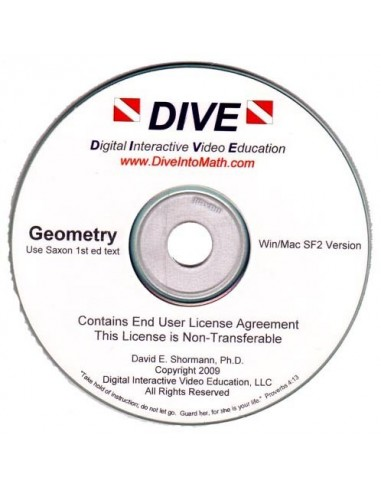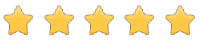See inside this book

# Geometry (1st ed) DIVE Into Math CD

\$60.00
No tax
10
P-MACD-28
In StockRead the review
Average rating: 5/5 Number of reviews: 1

#### Description

From Dive Into Math, this Interactive CD is for use with the Saxon Geometry 1st edition textbook. The DIVE CD-ROM teaches each of the 120 lessons and 12 Investigations, plus all the Construction and Technology Labs found in the Saxon Geometry textbook. Each lecture is about 10-20 minutes long. Geometry includes all topics in a high school geometry course, including perspective, space, and dimension associated with practical and axiomatic geometry. Students learn how to apply and calculate measurements of lengths, heights, circumference, areas, and volumes. Saxon Geometry introduces trigonometry and allows students to work with transformations. Students will use logic to create proofs and constructions and will work with key geometry theorems and proofs. Students use technology such as spreadsheets, graphing calculators, and geometry software to explore and understand geometry. Students watch the DIVE lecture and take notes, working the practice problems Dr. Shormann presents, pausing and rewinding as necessary until a concept is understood. If students want additional practice, they can complete the Example problems and Practice Set in the Saxon textbook. After the lesson, students complete the 30-question Problem Set in the Saxon text. While a few problems in the Problem Set come from the new concept, most problems come from previous lessons. Each problem is cross-referenced back to the lesson where it was taught, allowing students to quickly review any concept. Dr. Shormann explains how new topics connect to previously learned material, helping students see the "big picture". Also, when a student gets stuck or forgets how to work a problem, they simply click on the DIVE lecture where the concept was originally taught and watch that lesson again. All DIVE courses are taught from a Christian foundation. Dr. Shormann believes that God gave us mathematics primarily as a tool to study His creation, which is why mathematics is considered the language of science. Mathematics is also useful in interacting with each other and in developing reasoning and problem solving skills. Dr. Shormann uses Scripture to remind students that God is the author of all knowledge. Dr. Shormann also gives friendly reminders to students to honor their parents by doing excellent schoolwork. In DIVE Geometry, Dr. Shormann points out many important historical facts about geometry, and discusses many of the Christian mathematicians who used or discovered new ideas in geometry.

Please note that there are no returns or exchanges on software once the package or wrapping has been opened.

DIVE Into Math products are compatible with both Microsoft Windows and MAC.

10
Subject
Geometry
Math
Resources

### Reviews